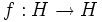# Extensibility-stable function property

This article defines a function metaproperty; a property that can be evaluated for properties of functions from a group to itselfTemplate:Formalism for function metaproperty

## Definition

### Symbol-free definition

A property$p$ of functions from a group to itself is said to be extensibility-stable if, given any subgroup of a group, and a function on the subgroup satisfying property$p$ in the subgroup, there is a function on the group satisfying property$p$ that extends the given function.

### Definition with symbols

A property$p$ of functions from a group to itself is said to be extensibility-stable if, given any groups$H \le G$, and a function$f: H \to H$ satisfying property$p$ in$H$, there is a function$f':G \to G$ such that$f'$ satisfies$p$ and such that the restriction of$f'$ to$H$ is$f$.

### In terms of the extensibility operator

Extensibility-stable function properties are precisely those function properties that are fixedp oints underthe extensibility operator on the function property space.

## Examples

### Inner automorphism

Any inner automorphism of a subgroup lifts to an inner automorphism of the whole group. This is because we can take the same conjugating element from the subgroup and use it to define a conjugation on the whole group. Note that since for a given inner automorphism, the choice of conjugating element is not unique, the lift is in general not unique. For full proof, refer: Inner is extensibility-stable